• 本程序主要实现了二维傅里叶变换，其中先对图像矩阵进行预处理（即图像中心化），然后进行行傅里叶变换，再对其进行列变换，进行行列变换是调用自己写的一维傅里叶变换函数ImFFT实现的。程序输入为图像矩阵A，输出为...
• 注：将个带有噪音的时域信号转化为频域信号 clc,clear; figure, Hz=0.02;%周期；也可以说x轴的步长 Fs=1/Hz; %频率 %定义函数 x=0:Hz:10-1/50; y=5*sin(2*pi*15*x) + 10*sin(2*pi*20*x)+ 17*cos(2*pi*5*x);%此处...

注：将一个带有噪音的时域信号转化为频域信号

clc,clear;
figure,
Hz=0.02;%周期；也可以说x轴的步长
Fs=1/Hz; %频率

%定义函数
x=0:Hz:10-1/50;
y=5*sin(2*pi*15*x) + 10*sin(2*pi*20*x)+ 17*cos(2*pi*5*x);%此处可以添加噪音
y=y+10*randn(size(x));
%上面的参数定义好，下面的不需要修改

subplot(2,1,1);
plot(x,y);
title("6*sin(10*2*pi*t)");

Y = fft(y);
L=length(x); % x 轴数据的个数
P2 = abs(Y/L);  % Y 为傅里叶变换得到的结果  L为Y的长度
P1 = P2(1:L/2+1);   %从 P2 取出元素 的 1--(500/2)+1 长度的元素  即，取前一半+1 个元素
P1(1:end-1) = 2*P1(1:end-1);
f = Fs*(0:(L/2))/L;
subplot(2,1,2);
plot(f,P1) ;
title("fft");
xlabel('Hz');
ylabel('振幅');

注：上面一些函数说明：
randn（）函数：https://blog.csdn.net/minyeling/article/details/105741721.

展开全文matlab
• 离散傅里叶变换的公式如下： 在MATLAB中，可以直接使用函数库fft(X)对一维向量X做傅里叶变换，分析信号的组成。如下例子处理一维离散信号信号分析通过傅里叶变换，可以将实变信号f(t)分解成各个频率分量的线性叠加，...

傅里叶变换的应用涵盖了概率与统计、信号处理、量子力学和图像处理等学科。

离散傅里叶变换的公式如下：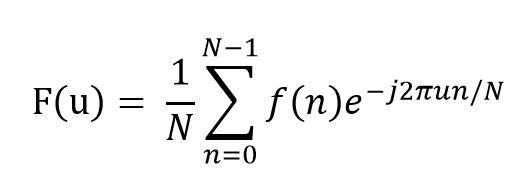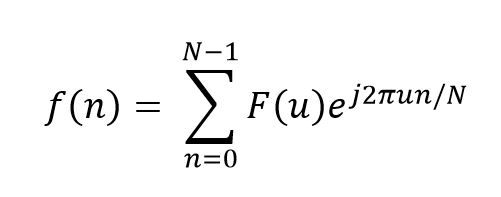在MATLAB中，可以直接使用函数库fft(X)对一维向量X做傅里叶变换，分析信号的组成。如下例子处理一维离散信号

信号分析

通过傅里叶变换，可以将实变信号f(t)分解成各个频率分量的线性叠加，进而从频率的角度研究信号的组成。

来看这个杂乱无章的曲线图，你是否能看出它的规律？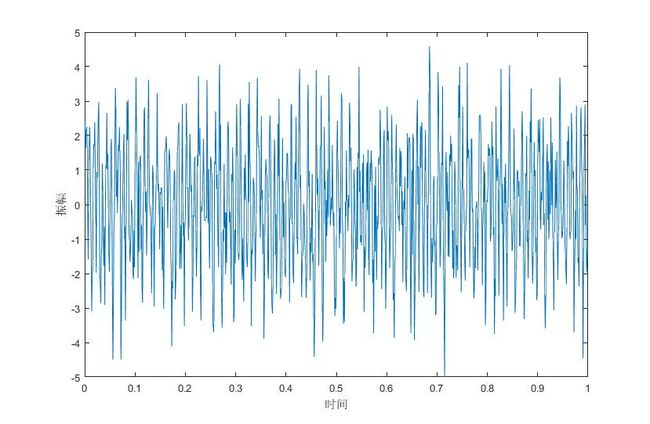Figure 1 What's the law of the signal?

让我们来对它做一维离散傅里叶变换，得到下图：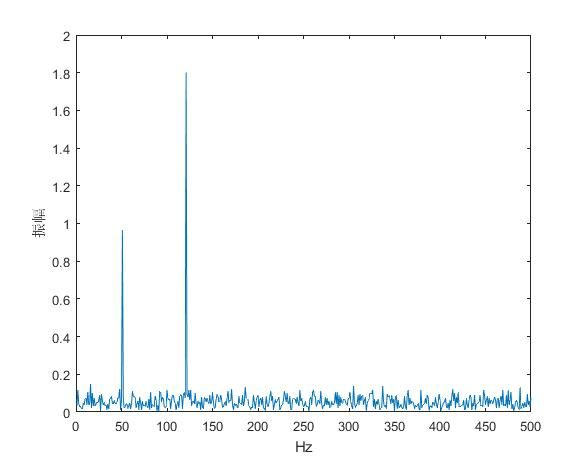Figure 2 The Fourier Transform of the signal in the figure 1

从图2的傅里叶变换中我们可以观察到信号在频率为50Hz和120Hz的地方有峰值，表明了原信号主要由频率为50Hz和120Hz的三角函数信号组成。

上面例子的具体MATLAB代码如下：

clear

Fs = 1000; % Sampling frequency

T = 1/Fs; % Sampling period

L = 1000; % Length of signal

t = (0:L-1)*T; % Time vector

S = 0.9*sin(2*pi*50*t) + 1.8*sin(2*pi*120*t); %standard signal

X = S + 1*randn(size(t)); %there are some noise mixing in the signal.

Y = fft(X); % Fourier Transform of the signal

f = 1:L/2; % the frequence of label

% half of Y is enough to show the information.

Y = Y./L*2; % calculate the amplitude of Fourier Transform of the signal

%draw the curve

figure(1);

plot(t,X); % show the original signal

xlabel('时间')

ylabel('振幅')

figure(2);

plot(f,abs(Y(1:L/2)));% show the Fourier Transform of the signal

xlabel('Hz');

ylabel('振幅');

傅里叶变换可以把信号的振动频率分离出来，我们可以通过设置不同频率分量前的系数，可实现信号的滤波。

如含有噪声的信号：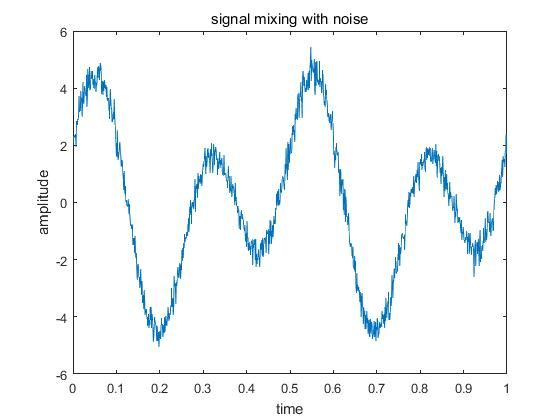Figure 3 signal mixing with noise

将该信号进行傅里叶变换，然后使得高频信号的系数为零，再傅里叶反变换回原信号，可得到滤波后的结果：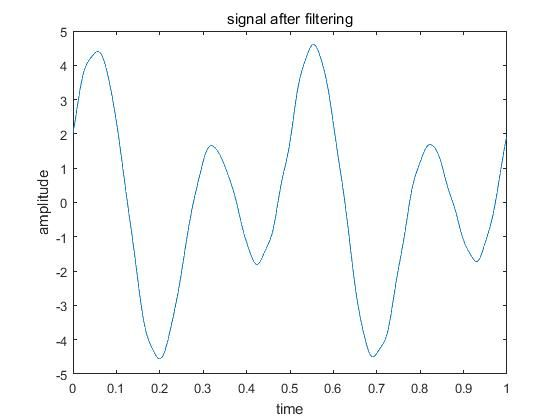Figure 4 signal after filtering

要注意的是，要想使用好傅里叶变换，必须对傅里叶变换有较为深入的了解，比如上面的滤波中，如果设置滤波频率的界限不同，那么其滤波效果也不同；还要注意的是，傅里叶变换后的信号具有周期性，其数据关于中心位置对称，所以滤波界限要关于中心对称；上例中具体实现代码如下：

clear

Fs = 1000;

T = 1./Fs;

L = 1000;

t = (0:L-1)*T;

S = 2*cos(2*pi*2*t)+3*sin(2*pi*4*t); %standard signal without the noise

X = S+0.3*randn(size(t)); %signal mixing with noise

figure(1);

plot(t,X);

title('signal mixing with noise');

xlabel('time');

ylabel('amplitude');

Y1 = fft(X); %fourier transform

X1 = ifft(Y1); %Inverse Fourier transform

figure(2);

plot(t,X1);

title('signal after filtering');

xlabel('time');

ylabel('amplitude');

本主要讲了离散傅里叶变换的两个应用——分析信号组成和滤波，其用处还很广泛，比如数据压缩、求微分方程等等。

展开全文• . 正弦函数分析 matlab代码如下： Fs = 1000; T = 1./Fs; L =1000; t = (0:L-1)*T; S0 = 2*cos(2*pi*2*t); figure(1),plot(t,S0); S00 = 3*cos(2*pi*4*t); figure(2),plot(t,S00); S1 = 2*cos(2*pi*2*t)+2*sin...

一. 正弦函数分析

matlab代码如下：

Fs = 1000;
T = 1./Fs;
L =1000;
t = (0:L-1)*T;
S0 = 2*cos(2*pi*2*t);
figure(1),plot(t,S0);
S00 = 3*cos(2*pi*4*t);
figure(2),plot(t,S00);
S1 = 2*cos(2*pi*2*t)+2*sin(2*pi*4*t);	%'b'
S2 = 2*cos(2*pi*2*t)+3*sin(2*pi*4*t);	%'r'
S3 = 2*cos(2*pi*2*t)+5*sin(2*pi*4*t);   %'g'
S4 = 4*cos(2*pi*2*t)+5*sin(2*pi*4*t);   %'k'
figure(3);title('original signal');
hold on,
plot(t,S1,'b');
plot(t,S2,'r');
plot(t,S3,'g');
plot(t,S4,'k');
hold off;

S1 = 2*cos(2*pi*2*t)+2*cos(2*pi*4*t);	%'b'
S2 = 2*cos(2*pi*2*t)+3*cos(2*pi*4*t);	%'r'
S3 = 2*cos(2*pi*2*t)+5*cos(2*pi*4*t);   %'g'
S4 = 4*cos(2*pi*2*t)+5*cos(2*pi*4*t);   %'k'
figure(4);title('original signal');
hold on,
plot(t,S1,'b');
plot(t,S2,'r');
plot(t,S3,'g');
plot(t,S4,'k');
hold off;

显示的图像如下：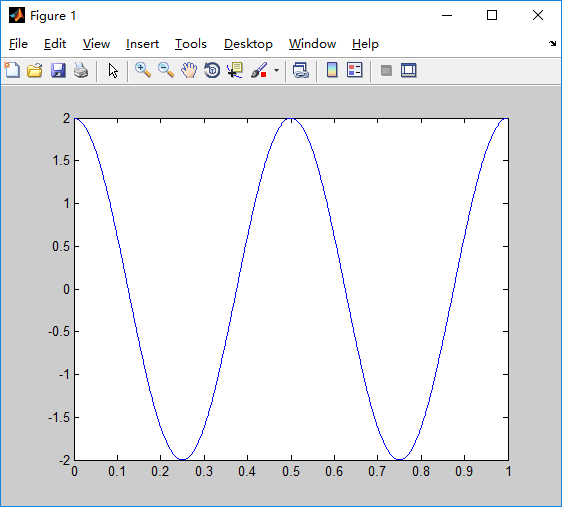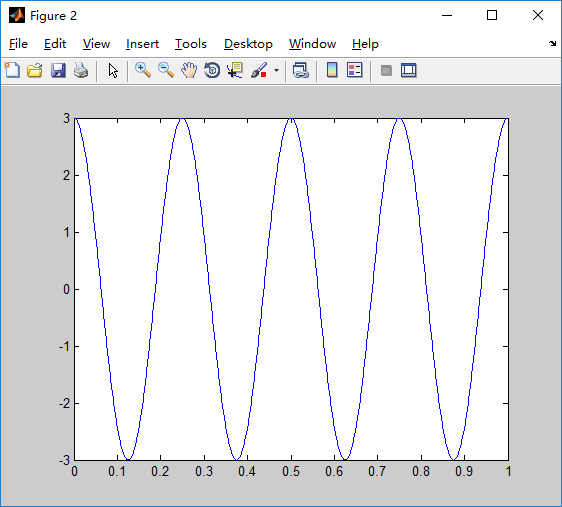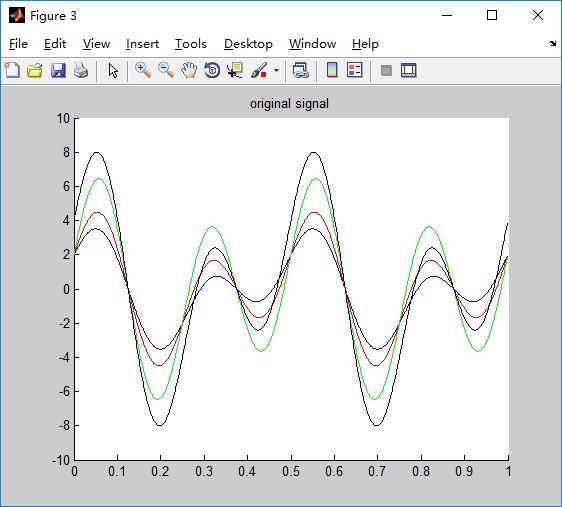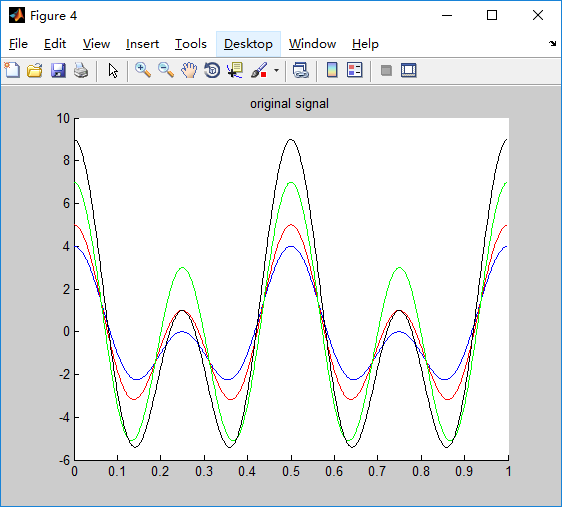Figure(1), S0的周期为0.5=（2*pi）/(2*pi*2)，频率为2Hz，最大幅值为2

Figure(2), S00的周期为0.25=（2*pi）/(2*pi*4)，频率为4Hz，最大幅值为3

Figure(3), 为一个正弦加上一个余弦函数，周期为0.5和0.25的最小公倍数，为0.5，最大幅值为8

Figure(4), 为两个余弦函数相加，周期为0.5和0.25的最小公倍数，为0.5，最大复制为9

总结：

1. 单一正弦函数，对应的最大幅值为sin函数前面的系数，周期和频率可以根据sin函数内部的定义计算得出。

2. 当周期变小，频率变大，曲线越密集。

3. 多个正弦函数相加，对应的周期为分别各个正弦函数的周期的最大公倍数，最大幅值要根据具体函数转换计算得到

二. 傅里叶变换

clear
clc
% close all

Fs = 1000;
T = 1./Fs;
L =1000;
t = (0:L-1)*T;

S0 = 2*sin(2*pi*20*t);    %standard signal without the noise
figure(1);
plot(t,S0);
title('original signal');
Y1 = fft(S0);                            %fourier transform
figure(2),plot(t,abs(Y1));

S = 2*sin(2*pi*20*t)+3*sin(2*pi*50*t);    %standard signal without the noise
figure(3);
plot(t,S);
title('original signal');
Y1 = fft(S);                            %fourier transform
figure(4),plot(t,abs(Y1));

S1 = 10+2*sin(2*pi*20*t)+3*sin(2*pi*50*t);    %standard signal without the noise
figure(5);
plot(t,S1);
title('original signal');
Y1 = fft(S1);                            %fourier transform
figure(6),plot(t,abs(Y1));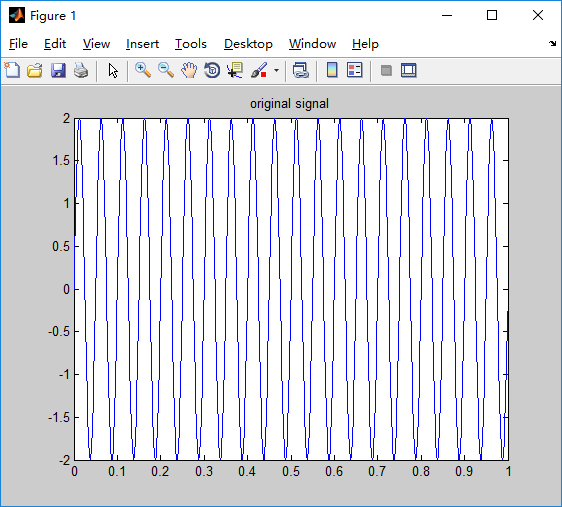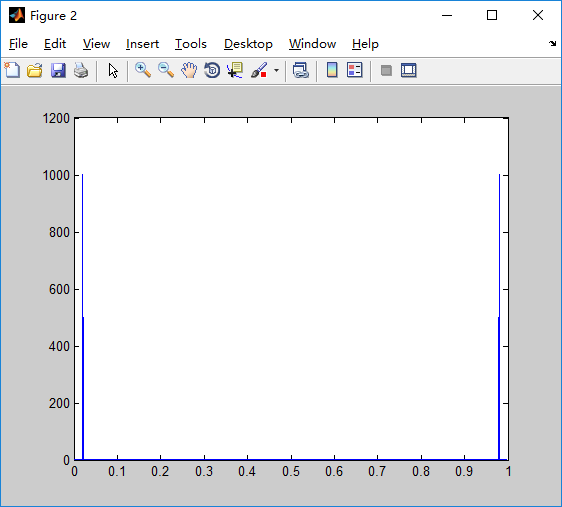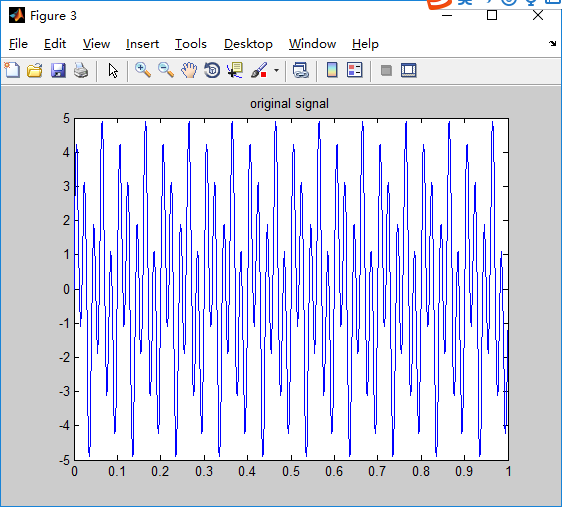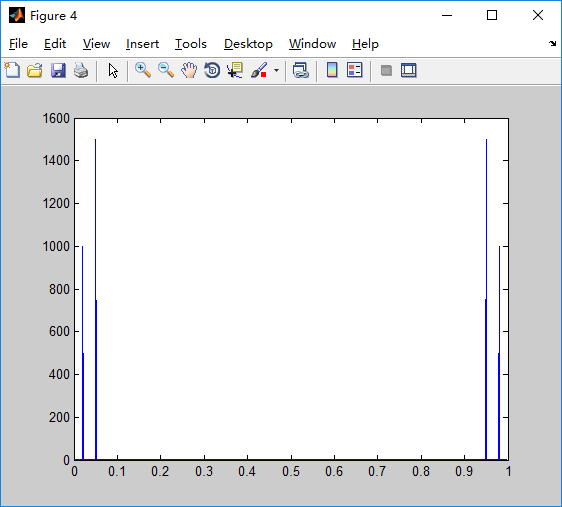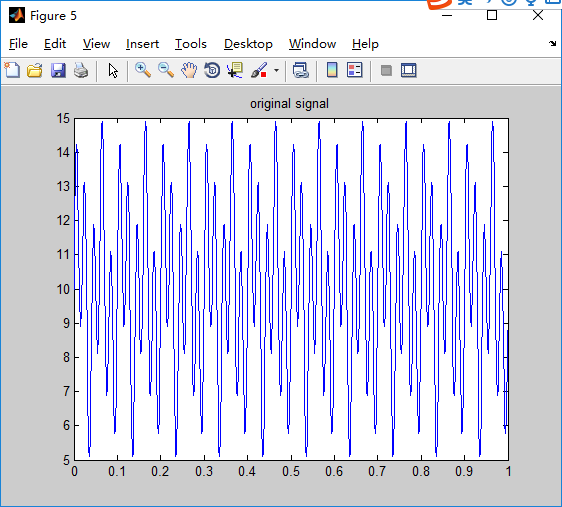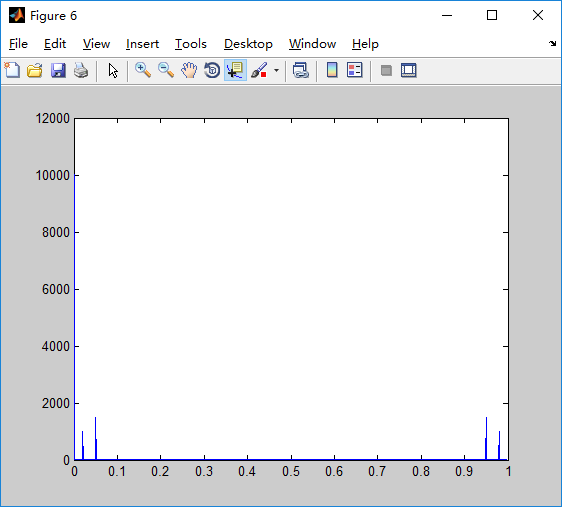Figure(1),Figure(2):单一正弦曲线和fft曲线；傅里叶曲线峰值为20/1000=0.02Hz(共轭为0.98Hz)，其它频率处的傅里叶值几乎为0

Figure(3),Figure(4):两个正弦曲线相加，以及对应的fft曲线；傅里叶曲线峰值有两个为20/1000=0.02Hz(共轭为0.98Hz)，50/1000=0.05Hz(共轭为0.95Hz)，其它频率处的幅值几乎为0

Figure(5),Figure(6):在两个正弦函数的基础上，增加了一个直流分量10；傅里叶曲线的峰值位置不变为20/1000=0.02Hz(共轭为0.98Hz)，50/1000=0.05Hz(共轭为0.95Hz)，在频率为0处增加了一个10*1000的直流分量线。

总结：

1. 傅里叶变换：将函数分解成直流分量+多个不同频率的正弦函数，然后进行傅里叶变换。

2. 单一正弦的傅里叶曲线，出现峰值的地方为频率所在的地方。

3. 多个正弦相加的傅里叶曲线，出现峰值的位置为两个频率所在的地方。

三. 噪声去噪

clear
clc
% close all

Fs = 1000;
T = 1./Fs;
L =1000;
t = (0:L-1)*T;

S1 = 2*sin(2*pi*2*t)+3*sin(2*pi*4*t);    %standard signal without the noise
X = S1+0.5*randn(size(t));               %signal mixing with noise
figure(1);
plot(t,X);
title('signal mixing with noise');xlabel('time');ylabel('amplitude');
Y = fft(X);                            %fourier transform

Y1 = Y;
X1 = ifft(Y1);                          %Inverse Fourier transform
figure(2);
plot(t,X1);
title('signal after filtering');xlabel('time');ylabel('amplitude');

Y2 = Y;
X2 = ifft(Y2);                          %Inverse Fourier transform
figure(3);
plot(t,X2);
title('signal after filtering');xlabel('time');ylabel('amplitude');

输出如下：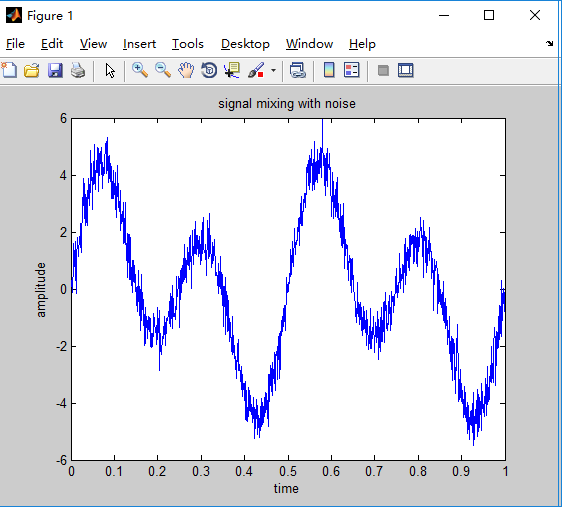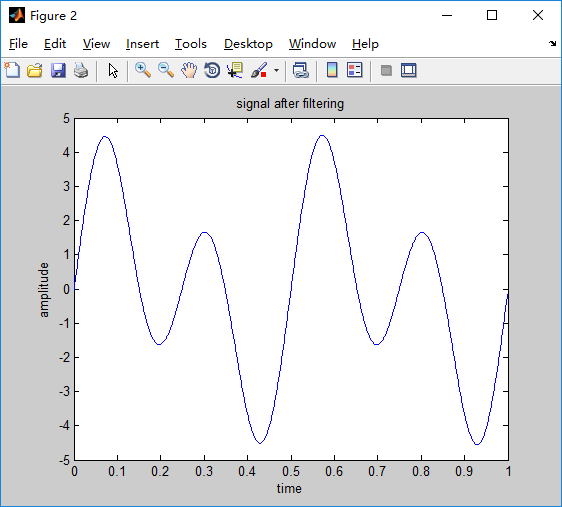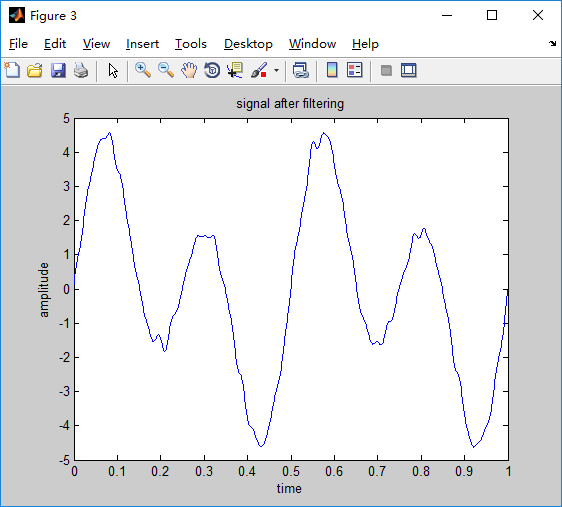总结：

通过傅里叶变换去除随机噪音，可以去除，但需要选择阈值不同，滤波效果不同

展开全文matlab 傅里叶变换
• 本程序是根据矩阵的形式实现了一维向量的快速傅里叶变换，程序输入为向量f，输出为其傅里叶变换所得序列F
• Y = fft(data1); L = size(data1,2); P2 = abs(Y/L); P1 = P2(1:L/2+1); P1(2:end-1) = 2*P1(2:end-1); Fs = 1000; f = Fs*(0:(L/2))/L; plot(f,P1);...title('Single-Sided Amplitude Spectrum of X(t)');...

Y = fft(data1);
L = size(data1,2);
P2 = abs(Y/L);
P1 = P2(1:L/2+1);
P1(2:end-1) = 2*P1(2:end-1);

Fs = 1000;
f = Fs*(0:(L/2))/L;
plot(f,P1);
title('Single-Sided Amplitude Spectrum of X(t)');
xlabel('f (Hz)');
ylabel('|P1(f)|');

展开全文• 原始数据如何转化成MATLAB可以识别的数据类型算法
• 如何画出分数阶傅里叶变换的三图像？我现在只能画出二图像来，我想把他拼成三图像，包含U域现在已有的代码是：function Faf = frft1(f, a)% The fast Fractional Fourier Transform% input: f = samples of ...
• % 对中心化变换后的图像求二维傅里叶变换 figure,imshow(imt_f,[]); title('使用自编函数fftcenter()中心变换图的二维傅里叶图谱'); mod_imt = abs(imt_f); % 要求幅度谱，需对傅里叶变换求模 mod_imt_log = uint8...
• 用matlab对图像进行二维傅里叶变换

千次阅读 多人点赞 2019-04-27 21:55:16
%进行二维傅里叶变换 F=fftshift(F); %对傅里叶变换后的图像进行象限转换 F=abs(F); %求傅里叶变换的模，我们都知道傅里叶变换后的结果为复数，包含real实部和imag虚部，  %abs就是求复数的模，经过这一步...matlab 二维傅里叶变换
• 回顾一下一维FT公式： 通俗来讲，一维傅里叶变换是将一个一维的信号分解成若干个复指数波。而由于 ，所以可以将每一个复指数波 都视为是 余弦波+j*正弦波的组合。对于一个正弦波而言，需要三...
• 没有调用matlab自带的fft函数，而是自己编写的二快速傅里叶变换fft程序 matlab平台 没有调用matlab自带的fft函数，而是自己编写的二快速傅里叶变换fft程序 matlab平台
• 1、傅里叶变换的幅值称为傅里叶谱或频谱。 2、F(u)的零值位置与“盒状”函数的宽度W成反比。 3、卷积定理：空间域两个函数的卷积的傅里叶变换等于两个函数的傅里叶变换在频率域中的乘积。f(t)*h(t) <=> H(u)F...
• 二维离散傅里叶变换matlab

万次阅读 2018-10-17 23:21:23
实际上对图像进行二维傅里叶变换得到频谱图，就是图像梯度的分布图，傅里叶频谱图上看到的明暗不一的亮点就是某一点与领域差异的强弱，即梯度的大小（该点的频率大小）。 .原理 1、代数形式的傅里叶变换： 2...
• 说明： Y = fft2(X) 使用快速傅里叶变换算法返回矩阵的二维傅里叶变换，这等同于计算 fft(fft(X).’).’。如果 X 是个多维数组，fft2 将采用高于 2 的每个维度的二维变换。输出 Y 的大小与 X 相同。 Y = fft2(X,m,...matlab
• 这学期选了《图像工程基础》这门课，课上老师留了个作业：对图像进行二维傅里叶变换。 现在我使用matlab解决这个问题 1.实验基本指令 首先我试了一下matlab图像处理的基本指令 原图： 经过以下指令后 将...
• 我们已经知道一维噪声可以用一维傅里叶变换到频域滤波，同理二维噪声也可以用二维傅里叶变换到"频率滤波"。 二维傅里叶正变换的原理 笔者很讨厌一上来就看到一连串复杂的公式！因此当我看懂一个原理后，我就会用最好...matlab
• 实验4 图像的二维傅里叶变换和频谱一、实验目的通过本实验使学生掌握使用MATLAB 进行二维傅里叶变换的方法，加深对二维傅里叶变换的理解和...实验用到的基本函数：一维傅里叶变换函数： fft,一维傅里叶变换函数...
• 话题：用MATLAB如何实现傅里叶变换问题详情：任意输入个函数,然后,输出函数的傅里叶变换函数,然后回答：x=sin(2*pi*t);%任意输入个函数 y=fft(x);%傅里叶变换函数 plot(abs(y));%振幅频率话题：matlab编程实现傅...
• Matlab中二维离散傅里叶变换

千次阅读 2020-06-09 09:48:55
FFT是快速傅里叶变换 中值滤波的理解： 还有种非线性滤波-中值滤波器。中值滤波器对脉冲型噪声有很好的去掉。因为脉冲点都是突变的点，排序以后输出中值，那么那些最大点和最小点就可以去掉了。中值滤波对高斯噪音...信号处理
• matlab的傅里叶变换

万次阅读 2019-10-18 14:18:29
更多MATLAB图像处理视频请点击  ...   图像变换：     更多MATLAB图像处理视频请点击  http://study.163.com/course/courseMain.htm?...matlab 傅里叶变换
• 汉宁窗傅里叶变换matlab代码Matlab音频过滤 为了充分了解设备的性能，还必须在频域中分析信号。 这正是频谱分析仪的工作。 但是，应该指出的是，随着数字技术的飞速发展，这种区别变得越来越模糊。 一些示波器可以...
• 关于二DFT的原理的话，可以参考清华出版的《图像工程 上册》，所以此处赘述二DFT的原理，如果不懂的话，那效果图像也不会理解为什么。 所以我在代码中直接调用库fft2(X); % by keyhero % img_fft.m clear; ...
• FINUFFT是一组库，用于在多核共享内存计算机上以一维，二维或三维有效地计算三种类型的非均匀快速傅里叶变换（NUFFT），以达到指定的精度。该库具有非常简单的界面，不需要任何预计算步骤，是用C ++（使用OpenMP和...
• http://www.doczj.com/doc/dafc8a4a82d049649b6648d7c1c708a1294a0aed.html用MATLAB制作二元傅里叶变换计算全息图作者：王永瑛张光明姜先策吴娜娜来源：《商情》2010年第18期[摘要]计算全息技术作为现代光学技术的...
• matlab中对图像进行傅里叶变换和逆变换。注意：m文件中的文件路径是在本人电脑上的路径，用户应修改成在您电脑中图片的绝对路径。谢谢大家的支持！
• 文章目录要求、连续函数抽样二、抽样长度确定三、DFT1、使用次循环实现DFT2、使用矩阵实现DFT总结 要求 有单频信号y(t)=sin(2πft)，其中f=100Hz，和f=500Hz，分别用DFT求y(t)的谱。  抽样频率fs以不发生...matlab 傅立叶分析
• 2020/3/3更新，增加了一些公式...回顾一下一维FT公式： 通俗来讲，一维傅里叶变换是将一个一维的信号分解成若干个复指数波 。而由于 ，所以可以将每一个复指数波 都视为是余弦波+j*正弦波的组合。对于一个正弦波而言...二维小波变换
• 实验题目 书上P274页找两张图求其的二维傅里叶变换的图像模 相位模*0相位1*相位第张的相位*第二张的模 二 算法说明 利用matlab对图像进行fft2,fftshift,abs,angle, Ifftshift,iff2t)等系列函数变换得到想要...
• A=fft2(double(I)); figure,imshow(A); %取傅里叶变换的模的平方； sfftI3=fftshift(A); %直流分量移到频谱中心 RR3=real(sfftI3); %取傅立叶变换的实部 II3=imag(sfftI3); %取......

matlab一维傅里叶变换matlab 订阅